﻿ Power Losses and Temperature Variations in a Power Converter for an Electronic Power Steering System Considering Steering Profiles

### Power Losses and Temperature Variations in a Power Converter for an Electronic Power Steering System...

Yong-feng Guo, Xin-lei Ma, Ping ShiOPEN ACCESSPEER-REVIEWED

## Power Losses and Temperature Variations in a Power Converter for an Electronic Power Steering System Considering Steering Profiles

Yong-feng Guo1,, Xin-lei Ma1, Ping Shi1

1School of Mechatronics Engineering, Harbin Institute of Technology, 150001 Harbin, China

### Abstract

Estimates of the lifespans of components in the power converter of electronic power steering (EPS) system are necessary for driving safety and comfort, and require accurate predictions of temperatures and power losses. Temperature profiles, including steady-state and transient conditions, are difficult to measure in actual vehicle operations. This study investigates a method to accurately calculate the power losses in the converter in an EPS system based on measured automobile steering cycles, the driving profile, and the currents and voltages of the semiconductor devices. In addition, the temperature variations in the electronic components are studied. This methodology is based on the relationship between temperature cycles and thermal resistance. The power loss and temperature model, which is implemented in MATLAB/Simulink, allows for the simulation of various power device losses, temperature and thermal resistances variations. The method is then verified for an EPS power converter. The power losses and temperature variations in the electronic components can be calculated, and these calculate values can be used to predict the reliability of the EPS system.

1234
Prev Next

### Cite this article:

• Guo, Yong-feng, Xin-lei Ma, and Ping Shi. "Power Losses and Temperature Variations in a Power Converter for an Electronic Power Steering System Considering Steering Profiles." Journal of Instrumentation Technology 2.1 (2014): 40-46.
• Guo, Y. , Ma, X. , & Shi, P. (2014). Power Losses and Temperature Variations in a Power Converter for an Electronic Power Steering System Considering Steering Profiles. Journal of Instrumentation Technology, 2(1), 40-46.
• Guo, Yong-feng, Xin-lei Ma, and Ping Shi. "Power Losses and Temperature Variations in a Power Converter for an Electronic Power Steering System Considering Steering Profiles." Journal of Instrumentation Technology 2, no. 1 (2014): 40-46.

 Import into BibTeX Import into EndNote Import into RefMan Import into RefWorks

### 1. Introduction

The use of electronic power steering (EPS) system in automobiles has received increased attention because EPS can reduce energy consumption, increase manoeuvrability, and enable intelligent driver-assistance systems compared to traditional power steering system. The EPS controller is typically mounted in the engine compartment, which is a high-temperature environment. Research has shown that large temperature cycles reduce the life of electronic components, which increases the failure rate of electronic systems. Accurate information on the temperature cycles of electronic components are required to ensure safety and reliability of vehicle. However, it is expensive and difficult to measure the temperature of electronic components. Hence, power loss and temperature estimation methods are required to solve these problems [4, 5].

Several methods have been proposed to improve the accuracy of power loss estimates. A general method for estimating power losses in Metal Oxide Semiconductor Field Effect Transistor (MOSFET) relies on datasheets and loss tables that include periodic pulses and hysteresis. A model of the circuit and the parameters of the components is required to compute power losses simply, accurately, and quickly . The switching power loss mechanisms can be used to calculate the power losses in a circuit [7, 8]. The computation time for power losses could be reduced by simplifying the calculations. A fast method for calculating power losses for a real-time thermal simulation of an Insulated Gate Bipolar Translator (IGBT) module was obtained by simplifying the system model [9, 10, 11]. The operation profile of electronic is crucial. Therefore, in this study, the vehicle steering profile is the actual data source for calculating the power losses of the components.

The finite element method (FEM) has been used to model the thermal behavior of circuits through the use of an equivalent circuit and discretisation of the heat equation. This method can be used to provide complete electro-thermal models to simulate a circuit, but the computational requirements are unacceptably large [12, 13]. An equivalent RC-network can be used to simplify the thermal analysis of the system and calculate the thermal cycle [14, 15]. This method assumes the steady state, but transient thermal cycles are also important. For transient behaviour, a dynamic electro-thermal model that depends on the instantaneous electrical power loss behaviour has been proposed to simulate semiconductor devices [16, 17]. The electro-thermal model for power losses was obtained by analytical methods. This model depends on the sub-microsecond switching characteristics of the devices, and appropriate parameters for the differential equations were selected to represent the thermal behavior. Research has revealed that temperature cycles are one of the most common causes of failures in electronic systems [18, 19].

In this study, the ambient conditions in the engine compartment of an automobile and the steering loads were obtained using the vehicle steering profile. The current, the voltage and steering torque were extracted from the profile and used to calculate the power losses. A novel method for calculating power losses based on the variation in resistance with temperature was developed. In addition, a method to calculate the power losses in the copper traces on printed circuit boards (PCBs) using the relationship between resistance and temperature with current was developed. The proposed temperature cycle estimation method is based on the power losses and the resistance model calculations. This method includes both steady-state and transient thermal conditions. The study of thermal cycles and power losses is crucial for predicting the lifespan of automobile EPS systems.

### 2. Simplification and Extraction of the Converter Profile

Accuracy and speed are two crucial factors for computations. To save time, the model of the EPS converter is simplified for calculating the power losses in the converter. First, components that consume small amounts of power are neglected in the model. The second step is to model the motor. The motor current is approximately constant, and Figure 1 illustrates that fluctuations in the current can be approximated with a smooth curve representing the large trend. The H-bridge currents and voltages are recorded in each switching cycle, and these data are used to formulate the power dissipation model of the EPS system. Without simplifications, it would be impossible to calculate an entire profile with all of the data and obtain accurate and reasonable parameters.

Figure 1. Actual and approximate converter current vs. input steering load

In the following steering profile, the current varies with the steering load in every cycle; the battery voltage drop is neglected. The converter generates the appropriate current for the steering load. In Figure 2(a), the value of the steering load was measured using a two-channels signal from a torque sensor, and in Figure 2(b), C1 and C2 are the two motor currents signals, which could be computed using the control law; the control law is computed using the torque variation.

### 3. Calculation of Converter Power Losses

In the EPS system, the power losses in the power electronic components are affected by factors such as the voltage, current, and ambient temperature. The converter dissipates the most power of all components in the EPS system. Parameters such as the voltage, current and resistance are provided by the steering profile. The ambient temperature, which influences the junction resistance, must be introduced in the power loss calculation and simulation. The power dissipation in the EPS system can be classified as either conduction losses or switching losses depending on the resistors, capacitors, and semiconductor devices.

3.1. Conduction Power Losses

Conduction power losses cannot be eliminated because of the equivalent resistances of the components. Source of conduction dissipation include the equivalent resistance in power devices, the resistance of the PCB copper traces and equivalent series resistance (ESR) of capacitors. The switching current can be assumed to be a constant (i.e., an average value) in the computations. To simplify the calculation and the simulation model, a reasonable approximation of the conduction dissipation can be computed using Equation (1), which describes the conduction dissipation for a MOSFET with a conductive resistance Rds ,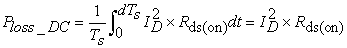(1)

where Ploss_DC is the power dissipation, Ts is the conduction times, ID is the continuous drain current, and Rds is the drain-to-source on-resistance. This equation is based on the assumptions that the switching times of the diodes and MOSFETs are negligible, the junction temperature is constant, the modulation of the H-bridge is linear, and the switching frequency fs is sufficiently fast.

The most important component in the converter is the MOSFET. The conduction resistances vary with the junction temperature, when the MOSFET operates for a long period of time . The conduction resistance varies with the junction temperature, and this variation can be approximated by Equation (2). The algorithm for computing the MOSFET conduction dissipation is illustrated in Figure 3. When the heat from power losses causes the conduction resistance to vary, the relative power dissipation will be affected in the next computation cycle. The power losses can be computed accurately using the properties of thermal variations in resistance and approximation formula.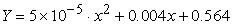(2)

The power losses vary with the resistance, and temperature changes affect the conduction resistance. The conduction dissipation will be greater than it would be for constant conduction resistances.

3.2. Switching Power Losses

Switching losses occur in electronic components due to imperfections in manufacturing and the properties of metals. There are several approaches to precisely simulate and detect switching losses in MOSFETs . Although some of these methods can produce good results, they are unsuitable for use in a long-duration driving profile. The computational process in this study use datasheets for the components provided by the manufactures. Because the EPS steering motor torque is an inductive load, there is an after-flow in the switching process. The switching dissipation in a MOSFET occurs both when the device switches on and when it switches off. The turn-on power loss can be calculated using Equation (3), where the voltage remains constant but the charging current increase, and the turn-off power loss is given by Equation (4), where the voltage varies but the current remains constant.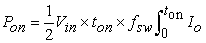(3)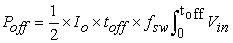(4)

In Equations (3) and (4),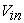is the battery voltage,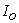is the conduction current,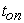is the turn-on time,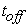is the turn-off time,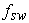is the switching frequency,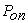is the turn-on power losses,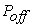is the turn-off power losses. The parasitic effects that exist in each pin of the MOSFET and are include in the model of the MOSFET are shown in Figure 4, where Cgd, Cgs and Cds are the gate to drain, gate to source, and drain to source parasitic capacitances, respectively.

The switching power losses can be calculated and distributed over the switching period based on the motor loads. The calculated total switching power losses are relatively small at approximately 0.032W. The switching losses are less than 1%, considerably less than the conduction losses, and the temperature increase is sufficiently small that the switching losses can be neglected.

3.3. Conduction Losses in PCB Copper Traces

Power flows from the battery to the motor through the PCB copper traces in the EPS converter. The equivalent resistance of the traces cannot be neglected because of the large currents. The total volume of the converter is restricted, so the area of the copper traces cannot be large. The EPS converter PCB wiring layout is depicted in Figure 5(a), in which the finite element mesh is also shown. The equivalent resistance is calculated using the FEM. The approximate formula in Equation (5) can be used to compute the resistance for an arbitrary shape of the trace layout ,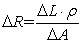(5)

where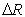is the equivalent resistance,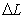is the copper pour element length,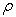is the resistivity, and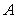is the element cross-sectional area.

When current flows through the copper traces, the decrease in voltage and the equivalent resistance cause energy dissipation. The power consumption causes a temperature increase in the PCB that further influences the resistance. The PCB temperature may increase considerably when the assist motor transient current or overload current exceeds its design value. The values of the motor current and ambient temperature are inputs to the simulation, whereas the current and temperature variation properties are known. The ambient temperature is extremely high because the EPS controller volume is extremely small and the controller is installed in the engine compartment.

### 4. Thermal Analysis and Modeling

A general method for computing the thermal behaviour of various geometries is the use of FEMs. Although these methods are extremely precise, the models are complicated and require a large number of calculations . Building an electrical analogue to calculate the temperature of the electronic components is the simplest method and is the method used in this study. In the EPS converter, the heat generated by the internal components is transferred to the air by conduction, convection, and radiation. The heat transfer is given by Equation (6) and Equation (7) provides the thermal resistance representing the thermal resistance between two solids in contact.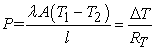(6)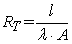(7)

In Equation (6) and (7), RT is the thermal resistance,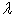is the thermal conductivity,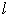is the conduction distance, andis the vertical cross-sectional area of the transfer path. The thermal dissipation of the EPS system can be obtained for both steady-state and transient conditions. Conduction and convection are coupled in the heat dissipation calculations.

4.1. Steady-state Temperature Analysis

The MOSFETs are the components that consume the most power in the EPS converter. The heat from the components is transferred from the junction to the heat sink and spreads out along the heat sink surface. The heat is then transferred from the cooling fins to surrounding air. The heat transfer path is shown in Figure 6 (a). Therefore, the conduction resistance is a crucial parameter for heat transfer because the heat sink provides only slight in heat dissipation, when the area of the heat sinks over wide surfaced are limited .

Using the resistance between the MOSFETs and aluminium heat sink, the heat transfer path can be modeled, and the specific thermal conductivity can be determined according to the material properties. The convection heat transfer coefficient h depends on the boundary conditions and must be calculated in a different manner. The heat sink convection is determined with the equivalent thermal resistance Rf used when calculating the heat transfer. The component power losses and temperature variations can be obtained using Equations (6) and (7).

The EPS converter supplies current from the H-bridge through the copper pours as the driver steers. However, the size of the EPS converter is extremely small because of the restricted mounting space. The heat generated by the MOSFET is transferred to the copper traces though the path shown in Figure 6(b). The equivalent thermal resistance network depends on the EPS H-bridge circuit illustrated in Figure 6(c), where Ri is the junction-to-case resistance, Rb is the junction-to-ambient resistance, Rs is the case-to-copper resistance, Rf1 is the copper-to-air resistance, Rc is the copper-to-PCB resistance, and Rf2 is the PCB-to-air resistance.

It is difficult to accurately calculate the values of the thermal resistance to convection between the aluminium sinks and ambient air. Equation (8) provides an approximation for calculating the resistance to convection, where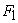is the contact area and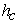is the heat transfer coefficient.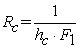(8)

Although this is a coupled and complex procedure, the steady-state convection heat transfer can be computed from Equation (9),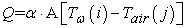(9)

where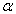is the convection heat transfer coefficient,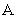is the convection surface area,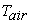is the sum of the atmospheric temperature and the engine compartment temperature, and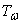is surface temperature. In the convection computations, the value of the heat transfer coefficientshould be confirmed first.

4.2. Transient heat transfer and temperature variations

Thermal energy cannot be transferred instantly because of thermal capacitance. The transfer time is related to the heat capacity, so the thermal loss time is constant. When the time t is sufficiently small, Eq. (10) is an effective approximation, where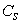is the heat capacity of the thermal transfer path and can be calculated from Equation (11). Equation (12) is used to calculate the increase in transient junction temperature. The equivalent electrical circuit for the transient case is illustrate in Figure 7(a). The temperature variation induced by the transient heat transfer and the single-pulse power loss are shown in Figure 7 (b).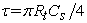(10)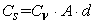(11)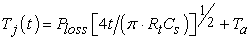(12)

When starting and in emergency steering, there are many changes in power consumptions. Because the time required for heat transfer is longer than the transition time, the junction temperature will increase rapidly. Equation (13) provides an approximate solution used in the simulation model (the coefficients can be obtained from the manufacture’s datasheet):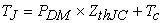(13)

where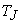is the junction temperature,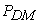is the transient power loss,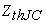is the transient thermal resistance, and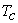is the ambient temperature. The transient pulse power is an important factor for calculating the transient temperature. The transient single-pulse power loss can be obtained from the manufacture’s datasheet and the operating procedures.

### 5. Results and Discussion

The effectiveness of the proposed power loss calculation method was tested in simulations. The EPS power losses vary with resistance, and the results indicate that the power losses with variations in resistance are different from those in which the resistances are constant, as shown in Figure 8. The A1 power loss curve in Figure 8 is for a fixed resistance, and the A2 power loss curve is for variable resistance. The power losses are larger in A2 than in A1. The temperature of the power device increased, which resulted in an increase in the resistance.

The simulation results illustrate that the switching losses were 0.032W and the conduction losses were approximately 1.5W with the proposed methodology. The EPS electronic system incurs losses from power devices and PCB copper traces. External factors include large temperature cycles in the vehicle engine compartment and variations in the atmospheric temperature due to weather. Figure 9 presents the relationship between current and temperature, while the resistances are constant.

In summary, when the resistance of the copper traces remains constant, the temperature increases with current. The thermal conduction model and equivalent thermal circuit have been validated. The results demonstrate that the proposed method for simulating the temperature cycles of the MOSFETs can model both the steady and transient temperatures and variations with the steering profile.

The power losses and temperature variations are shown in Figure 10, where T1 is the temperature, and P2 is the MOSFET power loss for the steering profile. The temperature variations correspond to the power losses. This figure illustrates the large fluctuations that occur while steering because the assist motor rapidly starts and stops. For the given steering profile, the simulation model produces a maximum transient temperature value in the MOSFETs of 110°C. The temperature cycle could be extracted from this figure.

The steering profile includes both steady-state and transient conditions. The duration of steering is only several seconds, which is longer than the thermal resistance time constant. Therefore, the heat transfer is not considered transient. However, the duration of the heat transfer is not sufficiently long to reach the steady state. In this condition, we should design mathematic method software to calculate and simulate the temperature accumulated in the next study. We plan to develop a method to calculate the temperature for this condition in future research.

### 6. Conclusions

This study investigated a novel method to model power losses in semiconductor devices in an automotive EPS system using the vehicle steering profile to provide certain calculation parameters. An analysis of the method indicated that the calculation parameters can be extracted from steering profile. MOSFETs are crucial power devices in an EPS system. Their power losses include switching losses and conduction losses, and the simulation results indicated that the switching losses amounted to 0.032W and the conduction losses amounted to approximately 1.5W. The results of the simulations indicated that the conduction resistance is related to the temperature variations, so power losses changed with every cycle. A temperature and resistance calculation scheme was proposed, and simulation results verified the method. An equivalent thermal network for the EPS converter was developed, and steady-state and transient heat transfer models were constructed. For a given steering profile, the power devices temperature was simulated, and this temperature profile could be used to predict the system reliability and estimate the lifespans of the electronic components.

### Acknowledgments

This study was supported by a grant from the Jiangsu Province Innovation Research Development Program of China.

### References

  Pou, J., Osorno, D., Zaragoza, J., Jaen, C. 'Power losses calculation methodology to evaluate inverter efficiency in electrical vehicles', Int. Conf. on Compatibility and Power Electronics., CPE, 2011, pp. 404-409.In article  Soeiro, T.B., Muhlethaler, J., Linner, J., Ranstad, P.: 'Automated Design of a High-Power High-Frequency LCC Resonant Converter for Electrostatic Precipitators', IEEE Trans. Ind. Electr., 2013, 60, (11), pp. 4805-4819.In article CrossRef  Costantino, N., Serventi, R., Tinfena, F., D'abramo, P.: 'Design and Test of an HV-CMOS Intelligent Power Switch With Integrated Protections and Self-Diagnostic for Harsh Automotive Applications', IEEE Trans. Ind. Electron., 2011, 58, (7), pp. 2715-2727.In article CrossRef  Yousef, F., Mohammad, T.B., Bahman, E.: 'Efficiency of three-level neutral-point clamped converters: analysis and experimental validation of power losses, thermal modelling and lifetime prediction', IET Power Electron., 2014, 7, (1), pp. 209-219.In article CrossRef  Takao, K., Ohashi, H.: 'Accurate Power Circuit Loss Estimation Method for Power Converters With Si-IGBT and SiC-Diode Hybrid Pair', IEEE Trans. Electron Devices., 2013, 60, (2), pp. 606-612.In article CrossRef  Bazzi, A.M., Krein, P.T., Kimbll, J.W.: 'IGBT and diode loss estimation under hysteresis switching', IEEE Trans. Power Electron., 2012, 27, (3), pp. 1044-1048.In article CrossRef  Chia-hsing, L., Kang, Y.L., Huang, J.CH., Tung, Y.CH.: 'Accurate power loss estimation for continuous current conduction mode synchronous Buck converters', Int. Conf. Anti-Counterfeiting, Security and Identification., ASID, 2012, pp. 1-5.In article  Testa, A., Caro, D.S, Russo, S.: 'A Reliability Model for Power MOSFETs Working in Avalanche Mode Based on an Experimental Temperature Distribution Analysis', IEEE Trans. Power Electron., 2012, 27, (6), pp. 2093-3100.In article CrossRef  Hirschmann, D., Tissen, D., Schroder, S., Dedoncker, R.W.: 'Inverter design for hybrid electrical vehicles considering mission profiles', Proc. IEEE Conf. Veh. Power Propulsion., 2006, pp. 567-573.In article  Casanellas, F.: 'Losses in PWM inverters using IGBTs', IEE Proc. Electron. Power Appl., 1994, 141, (5), pp. 235-239.In article  Garces, A., Molinas, M.: 'Comparative investigation of losses in a reduced matrix converter for off-shore wind turbines', Int. Conf. IET Power Electron, Machines and Drives., PEMD 2010, pp. 1-6.In article  Stabile, A., Boccaletti, C., Cardoso, A.J.M.: 'A Power Loss Measurement Method Applied to Static Power Converters', IEEE Trans. Instum. Meas, 2012, 62, (2), pp. 344-352.In article CrossRef  Huafeng, Xiao., Xipu, Liu., Ke, Lan.: 'Optimised full-bridge transformerless photovoltaic grid-connected inverter with low conduction loss and low leakage current', IET Power Electron., 2014, 7, (4), pp. 1008-1015.In article CrossRef  Wang, H., Khambadkone, A.M., Yu, X.: 'Control of parallel connected power converters for low voltage microgrid: Part II: Dynamic electrothermal modeling', IEEE Trans. Power Electron., 2010, 25, (12), pp. 2971-2980.In article CrossRef  Hari, V.S.S.P.K., Narayanan, G.: 'Space vector based hybrid PWM technique to reduce line current distortion in induction motor drives', IET Power Electron., 2012, 5, (8), pp. 1463-1471.In article CrossRef  Rajapakse, A.D., Gole, A.M., Wilson, P.L.: 'Electromagnetic transients simulation models for accurate representation of switching losses and thermal performance in power electronic systems', IEEE Trans. Power Del., 2005, 20, (1), pp. 319-325.In article CrossRef  Sheng, L., Zheng, X., Wen, H., Geng, T.: 'Electromechanical Transient Modeling of Modular Multilevel Converter Based Multi-Terminal HVDC Systems', IEEE Trans. Power Syst., 2014, 29, (1), pp. 72-83.In article CrossRef  Testa, A., Decaro, S., Russo, S.: 'A reliability model for power MOSFETs working in avalanche mode based on an experimental temperature distribution analysis', IEEE Trans. on Power Electrons., 2012, 27, (6), pp. 3093-3100.In article CrossRef  Bryant, A., Mawby, P., Palmer, P., Santi, E., Hudgins, J.: 'Exploration of power device reliability using compact device models and fast electro-thermal simulation', IEEE Trans. Ind. Appl., 2008, 44, (3), pp. 894-903.In article CrossRef  Agbrahe, O., Kavanagh, D.F., Sumislawska, M., Howey, D.A.: 'Estimation of temperature dependent equivalent circuit parameters for traction-based electric machines', Int. Conf. IET Hybrid and Electric Vehicles., HEVC 2013, pp. 4.3.In article  Blewitt, W.M., Atkinson, D.J., Kelly, J., Lakin, R.A.: 'Approach to low-cost prevention of DC injection in transformerless grid connected inverters', IET Power Electron., 2010, 3, (1): pp. 111-119.In article CrossRef  Chaturvedi, P., Jain, S., Agarwal, P.: 'Carrier-Based Neutral Point Potential Regulator With Reduced Switching Losses for Three Level Diode Clamped Inverter', IEEE Trans. Ind. Electr., 2014, 61, (2), pp. 613-624.In article CrossRef  Amy, R.A., Aglietti, G.S., Richardson, G.: 'Accuracy of simplified printed circuit board finite element models', Microelectronics Reliability., 2010, 50, pp. 86-97.In article CrossRef  Musallam, M., Johnson, C.M.: 'Real-Time Compact Thermal Models for Health Management of Power Electronics', IEEE Trans. Power Electron., 2010, 25, (6), 1416-1425.In article CrossRef  Jaldanki, S.S.P., Gopalaratnam, N.: 'Minimum switching loss pulse width modulation for reduced power conversion loss in reactive power compensators', IET Power Electron., 2014, 7, (3), 545-551.In article CrossRef